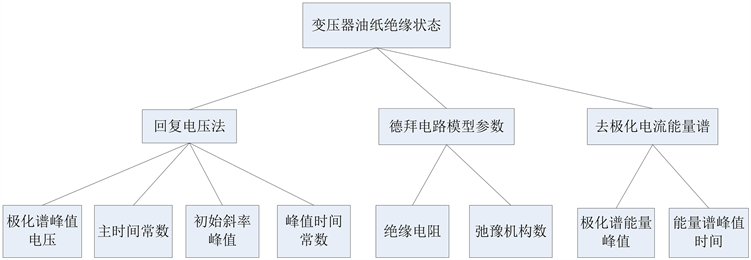﻿ 可拓理论在变压器油纸绝缘状态综合评估中的应用

# 可拓理论在变压器油纸绝缘状态综合评估中的应用Application of Extension Theory in Comprehensive Assessment of Transformer Oil-Paper Insulation

Abstract: Due to the fuzziness and uncertainty of the transformer’s insulation state by itself, when using the index to evaluate the oil-paper insulation transformers alone, it may appear contradictory and incompatible situations. In this paper, an evaluation model based on extension theory is proposed to comprehensively evaluate the oil paper insulation state of transformer by integrating multi time domain features. Firstly, the hierarchical framework of transformer evaluation is established, and the extension analytic hierarchy process is introduced to determine the weight coefficient of each index; Secondly, we need to build the classical domain and joint domain of transformer’s four kinds of matter-element models using large amounts of the measured data. Then, using correlation function to determine the insulation state of transformers, this paper realizes the synthetic evaluation with the qualitative and quantitative combination for the transformer. Finally, the effectiveness and feasibility of the method are verified by an example.

1. 引言

2. 可拓理论

2.1. 物元模型

$R=\left(\begin{array}{ccc}N& {c}_{1}& {v}_{1}\\ & {c}_{2}& {v}_{2}\\ & ⋮& ⋮\\ & {c}_{n}& {v}_{n}\end{array}\right)$ (1)

2.2. 物元的经典域与节域

${R}_{p}=\left(\begin{array}{ccccccc}N& {N}_{\text{1}}& {N}_{\text{2}}& \cdots & {N}_{j}& \cdots & {N}_{m}\\ {c}_{1}& {v}_{p\text{11}}& {v}_{p\text{11}}& \cdots & {v}_{p\text{1}j}& \cdots & {v}_{{}_{p1m}}\\ {c}_{2}& {v}_{p21}& {v}_{p22}& \cdots & {v}_{p2j}& \cdots & {v}_{p2m}\\ ⋮& ⋮& ⋮& \ddots & ⋮& \ddots & ⋮\\ {c}_{i}& {v}_{pi1}& {v}_{pi2}& \cdots & {v}_{pij}& \cdots & {v}_{pim}\\ ⋮& ⋮& ⋮& \ddots & ⋮& \ddots & ⋮\\ {c}_{n}& {v}_{pn1}& {v}_{pn2}& \cdots & {v}_{pnj}& \cdots & {v}_{pnm}\end{array}\right)$ (2)

$\left\{\begin{array}{l}{\mu }_{ij}=\frac{1}{{t}_{j}}\underset{k}{\overset{{t}_{j}}{\sum }}{v}_{ijk}\\ {\sigma }_{ij}=\sqrt{\frac{1}{{t}_{j}}\underset{k}{\overset{{t}_{j}}{\sum }}{\left({v}_{i}-{\mu }_{ij}\right)}^{2}}\end{array}$ (3)

${R}_{q}=\left(\begin{array}{ccccccc}N& {N}_{1}& {N}_{2}& \cdots & {N}_{j}& \cdots & {N}_{m}\\ {c}_{1}& {v}_{q11}& {v}_{q12}& \cdots & {v}_{q1j}& \cdots & {v}_{{}_{q1m}}\\ {c}_{2}& {v}_{q21}& {v}_{q22}& \cdots & {v}_{q2j}& \cdots & {v}_{q2m}\\ ⋮& ⋮& ⋮& \ddots & ⋮& \ddots & ⋮\\ {c}_{i}& {v}_{qi1}& {v}_{qi2}& \cdots & {v}_{qij}& \cdots & {v}_{qim}\\ ⋮& ⋮& ⋮& \ddots & ⋮& \ddots & ⋮\\ {c}_{n}& {v}_{qn1}& {v}_{qn2}& \cdots & {v}_{qnj}& \cdots & {v}_{qnm}\end{array}\right)$ (4)

${v}_{qij}=〈{v}_{ij\mathrm{min}},{v}_{ij\mathrm{max}}〉$vijmin表示特征值cij种状态下对应的特征值的最小值 ${v}_{ij\mathrm{min}}=\underset{k}{\overset{{t}_{j}}{\mathrm{min}}}\left({v}_{ijk}\right)$vijmax表示ci在第j种状态下对应的特征值的最大值 ${v}_{ij\mathrm{max}}=\underset{k}{\overset{{t}_{j}}{\mathrm{max}}}\left({v}_{ijk}\right)$

2.3. 关联函数

$K=\left\{\begin{array}{l}\frac{\rho \left({v}_{i},{v}_{pij}\right)}{\rho \left({v}_{i},{v}_{qij}\right)-\rho \left({v}_{i},{v}_{pij}\right)},\rho \left({v}_{i},{v}_{qij}\right)\ne \rho \left({v}_{i},{v}_{pij}\right)\\ -\rho \left({v}_{i},{v}_{pij}\right)-1,\rho \left({v}_{i},{v}_{qij}\right)=\rho \left({v}_{i},{v}_{pij}\right)\end{array}$ (5)

$\rho \left({v}_{i},{v}_{pij}\right)=|{v}_{i}-\frac{{v}_{pij1}+{v}_{pij2}}{2}|-\frac{{v}_{pij2}-{v}_{pij1}}{2}$ (6)

2.4. 可拓层次分析法确定指标权重

$i,j=\text{1},\text{2},\cdots ,n$ 均有： ${e}_{ij}=〈{e}_{ij}^{-},{e}_{ij}^{+}〉$ ，且 $1/9\le {e}_{ij}^{-}\le \text{9}$${e}_{ii}=\text{1}$${e}_{ji}={e}_{ij}^{-1}=〈1/{e}_{ij}^{+},1/{e}_{ij}^{-}〉$ 。各指标的权重的具体确定步骤如下：

1) 求出左判断矩阵 ${E}^{-}={\left({e}_{ij}^{-}\right)}_{n×n}$ 和右判断矩阵 ${E}^{+}={\left({e}_{ij}^{+}\right)}_{n×n}$ 的最大特征值分别对应特征向量，并对其进行归一化处理，得到 ${X}^{-}={\left({a}_{ij}^{-}\right)}_{n×n}$${X}^{+}={\left({a}_{ij}^{-}\right)}_{n×n}$

2) 求 $\lambda ={\left({\lambda }_{1},{\lambda }_{2},\cdots ,{\lambda }_{n}\right)}^{\text{T}}=〈r{X}^{-},s{X}^{+}〉$ ，其中rs由式(7)计算。

$\left\{\begin{array}{l}r=\sqrt{\underset{j=1}{\overset{n}{\sum }}\frac{1}{\underset{i=1}{\overset{n}{\sum }}{a}_{ij}^{+}}}\\ s=\sqrt{\underset{j=1}{\overset{n}{\sum }}\frac{1}{\underset{i=1}{\overset{n}{\sum }}{a}_{ij}^{-}}}\end{array}$ (7)

3) 通过λ求出各指标单层排序权重。

$V\left(e\ge f\right)=\frac{2\left({e}^{+}-{f}^{-}\right)}{\left({e}^{+}-{e}^{-}\right)+\left({f}^{+}-{f}^{-}\right)}$ (8)

4) 由式(8)可计算 $V\left({\lambda }_{i}\ge {\lambda }_{j}\right)$ ，( $i=1,2,\cdots ,n\ge 0$$i\ne j$ )。若 $V\left({\lambda }_{i}\ge {\lambda }_{j}\right)\ge 0$ ，则 ${\omega }_{j}=\text{1}$${\omega }_{i}=V\left({\lambda }_{i}\ge {\lambda }_{j}\right)$

3. 可拓理论在变压器油纸绝缘状态评估中的应用

3.1. 变压器可拓评估指标体系的建立Figure 1. Analytic hierarchy diagram of transformer’s evaluation index

${v}_{i}=\frac{{{v}^{\prime }}_{i}-{{v}^{\prime }}_{i\text{-}\mathrm{min}}}{{{v}^{\prime }}_{i\text{-}\mathrm{max}}-{{v}^{\prime }}_{i\text{-}\mathrm{min}}}$ (9)

${v}_{i}=\frac{{{v}^{\prime }}_{i\text{-}\mathrm{max}}-{{v}^{\prime }}_{i}}{{{v}^{\prime }}_{i\text{-}\mathrm{max}}-{{v}^{\prime }}_{i\text{-}\mathrm{min}}}$ (10)

3.2. 确定变压器各状态等级的经典域物元模型和节域物元模型

${R}_{p}=\left(\begin{array}{ccccc}N& {N}_{1}& {N}_{2}& {N}_{3}& {N}_{4}\\ {c}_{1}& 〈0.0266,0.0824〉& 〈0.2226,0.3258〉& 〈0.0723,0.5403〉& 〈0.3371,1〉\\ {c}_{2}& 〈0,0.1108〉& 〈0.3129,0.8511〉& 〈0.1937,0.9929〉& 〈0.9459,1〉\\ {c}_{3}& 〈0,0.6187〉& 〈0.3908,0.5528〉& 〈0.1868,1〉& 〈0.2987,1〉\\ {c}_{4}& 〈0,0.8517〉& 〈0.34,0.4528〉& 〈0.2802,0.7266〉& 〈0,1〉\\ {c}_{5}& 〈0,0.025〉& 〈0,1〉& 〈0,1〉& 〈0.0119,1〉\\ {c}_{6}& 〈0,0.1034〉& 〈0,0.8496〉& 〈0,1〉& 〈0.4646,1〉\\ {c}_{7}& 〈0,0.4428〉& 〈0,1〉& 〈0.1851,0.9033〉& 〈0.5,1〉\\ {c}_{8}& 〈0,0.1629〉& 〈0,0.8684〉& 〈0,1〉& 〈0.9732,1〉\end{array}\right)$

${R}_{q}=\left(\begin{array}{ccccc}N& {N}_{1}& {N}_{2}& {N}_{3}& {N}_{4}\\ {c}_{1}& 〈0.0232,0.1859〉& 〈0.1517,0.3933〉& 〈0.0723,0.5724〉& 〈0.2767,1〉\\ {c}_{2}& 〈0,0.1706〉& 〈0.2122,0.8912〉& 〈0.0024,0.9929〉& 〈0.9243,1〉\\ {c}_{3}& 〈0,0.7284〉& 〈0.2435,0.5743〉& 〈0.1272,1〉& 〈0.2264,1〉\\ {c}_{4}& 〈0,0.9327〉& 〈0.23,0.67〉& 〈0.2607,0.8327〉& 〈0,1〉\\ {c}_{5}& 〈0,0.2432〉& 〈0,1〉& 〈0,1〉& 〈0,1〉\\ {c}_{6}& 〈0,0.2023〉& 〈0,0.9322〉& 〈0,1〉& 〈0.4213,1〉\\ {c}_{7}& 〈0,0.6396〉& 〈0,1〉& 〈0,1〉& 〈0.5,1〉\\ {c}_{8}& 〈0,0.2736〉& 〈0,0.8722〉& 〈0,1〉& 〈0.9439,1〉\end{array}\right)$

3.3. 确定变压器各指标的权重

$A=\left(\begin{array}{ccc}〈1,1〉& 〈5,7〉& 〈3,4〉\\ 〈\frac{1}{7},\frac{1}{5}〉& 〈1,1〉& 〈\frac{1}{4},\frac{1}{3}〉\\ 〈\frac{1}{4},\frac{1}{3}〉& 〈3,4〉& 〈1,1〉\end{array}\right)$

${B}_{1}=\left(\begin{array}{cccc}〈1,1〉& 〈\frac{1}{7},\frac{1}{5}〉& 〈3,4〉& 〈5,7〉\\ 〈5,7〉& 〈1,1〉& 〈6,7〉& 〈7,9〉\\ 〈\frac{1}{4},\frac{1}{3}〉& 〈\frac{1}{7},\frac{1}{6}〉& 〈1,1〉& 〈3,4〉\\ 〈\frac{1}{7},\frac{1}{5}〉& 〈\frac{1}{9},\frac{1}{7}〉& 〈\frac{1}{4},\frac{1}{3}〉& 〈1,1〉\end{array}\right)$

${B}_{2}=\left(\begin{array}{cc}〈1,1〉& 〈\frac{1}{3},\frac{1}{2}〉\\ 〈2,3〉& 〈1,1〉\end{array}\right)$

${B}_{3}=\left(\begin{array}{cc}〈1,1〉& 〈\frac{1}{4},\frac{1}{3}〉\\ 〈3,4〉& 〈1,1〉\end{array}\right)$

3.4. 基于可拓理论的状态评估步骤

1) 按式(2)~(4)确定变压器各状态等级物元的经典域、节域及待评估变压器物元模型。

2) 按照可拓层次分析法的步骤确定各特征值权重。

3) 根据2.3节的关联函数，按式(5)计算各特征值关于绝缘状态等级的关联度K(vi)。

4) 结合确定的各指标权重系数wi，根据式(11)计算待评估物元N关于各状态等级的关联度Kj(N)。

${K}_{j}\left(N\right)=\underset{i=1}{\overset{8}{\sum }}{w}_{i}×K\left({v}_{i}\right)$(11)

5) 根据式(12)，确定出待评估变压器物元N的绝缘状态等级。

${K}_{{j}^{\prime }}=\mathrm{max}\left\{{K}_{j}\left(N\right)\right\},\text{\hspace{0.17em}}j=1,2,3,4$(12)

4. 实例分析Table 1. Statistical data of the measured values of 8 transformersTable 2. The correlation degree of 8 transformers on each state level

5. 结论

1) 运用可拓层次分析法求解变压器各个特征量的权重的过程，综合了多名专家的建议，构造可拓判断区间，有效地对变压器各参量进行赋权，同时将权重向量的求解与一致性检验有机结合起来，大大减少了传统层次分析法验证一致性过程的繁杂计算。

2) 将可拓理论中的物元模型和关联函数引入了变压器绝缘状态评估过程中，定量的计算变压器绝缘状况等级的同时能够融合多特征量进行评估，较单一特征量对变压器绝缘状态进行评估具有更高的精确度。

3) 把概率论中正态分布的理论运用到求解经典域和节域的过程当中，可以最大限度地利用历史参考数据库。

4) 将实测数据、实际绝缘状态与基于可拓理论的绝缘状态理论计算结果进行对比，其结果具有一致性，验证了基于可拓理论的变压器油纸绝缘状态综合评估方法的可行性、有效性及准确性。

 刘捷丰, 廖瑞金, 吕彦冬, 等. 电力变压器油纸绝缘含水量定量评估的时域介电特征量[J]. 电工技术学报, 2015, 30(2): 196-203．

 刘骥, 赵明云, 等. 变压器油纸绝缘老化介电谱特性及特征量研究[J]. 电机与控制学报, 2018, 22(11): 67-74.

 宋人杰, 刘瑞英, 王林. 灰色定权聚类和变权模式在变压器状态评估中的应用研究[J]. 电工电能新技术, 2017, 36(3): 75-80.

 贺德华, 蔡金锭, 黄云程. 基于等效电路参数特征量的油纸绝缘老化状态评估[J]. 电机与控制学报, 2017, 21(6): 44-49.

 廖瑞金, 王谦, 骆思佳, 等. 基于模糊综合评判的电力变压器运行状态评估模型[J]. 电力系统自动化, 2008, 32(3): 70-75.

 刘骥, 尹梦涵, 李秀婧, 等. 油纸绝缘老化评估的Davidson-Cole模型参数提取方法[J]. 电机与控制学报, 2017, 21(3): 63-70.

 蔡文, 杨春燕. 可拓学的基础理论与方法体系[J]. 科学通报, 2013, 58(13): 1190-1199.

 黄文涛, 赵学增, 王伟杰, 等. 基于物元可拓模型的电力变压器故障的可拓诊断方法[J]. 电力系统自动化, 2004, 28(13): 45-49.

 马丽叶, 丁荣荣, 卢志刚, 等. 基于可拓云模型的配电网经济运行综合评价及灵敏度分析[J]. 电工电能新技术, 2016, 35(7): 8-16.

 杨贵军, 蒋朝辉, 桂卫华, 等. 基于熵权-可拓理论的高炉软熔带位置状态模糊综合评判方法[J]. 自动化学报, 2015, 41(1): 75-83.

 廖瑞金, 张镱议, 黄飞龙, 等. 基于可拓分析法的电力变压器本体绝缘状态评估[J]. 高电压技术, 2012, 38(3): 521-526.

Top